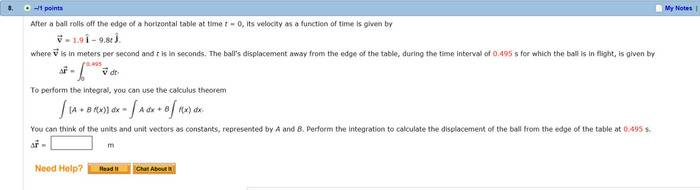# Integration of an equation to find displacement

## Homework StatementAbove

## The Attempt at a Solution

Okay, so I understand I need to integrate the top equation because it's velocity as a function of time.

I just don't know how.

So, what is $\int A \ dt$ ?